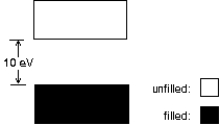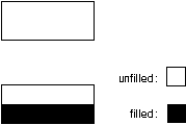# Fundamentals of Physics Study Set 2

Physics & Astronomy

## Quiz 41 :Conduction of Electricity in SolidsLooking for Introductory Physics Homework Help?

## Quiz 41 :Conduction of Electricity in Solids

Showing 1 - 20 of 49The Fermi-Dirac probability function P(E)varies between:
Free
Multiple Choice

AWe classify solids electrically according to three basic properties.What are they?
Free
Multiple Choice

DThe number density n of conduction electrons, the resistivity , and the temperature coefficient of resistivity α are given below for five materials.Which is a semiconductor?
Free
Multiple Choice

DA certain material has a resistivity of 7.8 103 m at room temperature, and its resistivity increases as the temperature is raised by 100C.The material is most likely:
Multiple ChoiceA certain material has a resistivity of 7.8 103 m at room temperature, and its resistivity decreases as the temperature is raised by 100C.The material is most likely:
Multiple ChoiceA certain material has a resistivity of 7.8 10-8 m at room temperature, and its resistivity increases as the temperature is raised by 100C.The material is most likely:
Multiple ChoiceWhich one of the following statements concerning electron energy bands in solids is true?
Multiple ChoiceIf E0 and ET are the average energies of the "free" electrons in a metal at 0 K and room temperature respectively, then the ratio ET/E0 is approximately:
Multiple ChoiceThe density of states for a metal depends primarily on:
Multiple ChoiceThe Fermi energy of a metal depends primarily on:
Multiple ChoiceThe energy gap between the valence and conduction bands of an insulator is of the order:
Multiple ChoiceThe energy level diagram shown applies to:Multiple ChoiceElectrons in a full band do not contribute to the current when an electric field exists in a solid because:
Multiple ChoiceThe energy level diagram shown applies to:Multiple ChoiceIn a metal at 0 K, the Fermi energy is:
Multiple ChoiceThe speed of an electron with energy equal to the Fermi energy for copper is on the order of:
Multiple ChoicePossible units for the density of states function N(E)are:
Multiple ChoiceAt T = 0 K the probability that a state 0.50 eV below the Fermi level is occupied is:
Multiple Choice